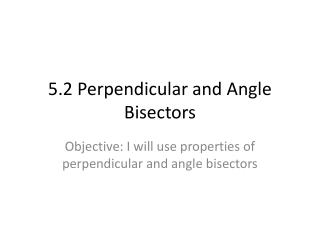Download Presentation5.2 Perpendicular and Angle Bisectors5.2 Perpendicular and Angle Bisectors - PowerPoint PPT Presentation

Download Presentation5.2 Perpendicular and Angle Bisectors
An Image/Link below is provided (as is) to download presentation

Download Policy: Content on the Website is provided to you AS IS for your information and personal use and may not be sold / licensed / shared on other websites without getting consent from its author. While downloading, if for some reason you are not able to download a presentation, the publisher may have deleted the file from their server.

- - - - - - - - - - - - - - - - - - - - - - - - - - - E N D - - - - - - - - - - - - - - - - - - - - - - - - - - -
Presentation Transcript

1. 5.2 Perpendicular and Angle Bisectors Objective: I will use properties of perpendicular and angle bisectors

2. Vocab 1. equidistant: a point that is the same distance from two objects 2. Distance from a point to a line: length of the perpendicular segment from the point on the line

3. Theorem 5-2: Perpendicular Bisector Theorem If a point in on the perpendicular bisector of a segment, then it is equidistant from the endpoints of the segment If: Then: PM I AB and MA = MBPA = PB P P B A M B A M

4. Theorem 5-3: Converse of the Perpendicular Bisector Theorem If a point is equidistant from the endpoints of a segment, then it is on the perpendicular bisector of the segment If: Then: PA = PB PM I AB and MA = MB P P B A M B A M

5. Theorem 5-4: Angle Bisector Theorem If a point is on the bisector of an angle, then the point is equidistant from the sides of the angle If: Then: QS bisects <PQR, SP I QP, SP = SR And SR I QR P P Q S Q S R R

6. Theorem 5-5: Converse of the Angle Bisector Theorem If a point in the interior of an angle is equidistant from the sides of the angle, then the point is on the angle bisector If: Then: SP = SR, SP I QP,QS bisects <PQR and SR I QR P P Q S Q S R R